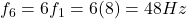## If a wave’s third harmonic has a frequency of 24 Hz, what is its natural (fundamental) frequency and what is the frequency of H6?

Question

If a wave’s third harmonic has a frequency of 24 Hz, what is its
natural (fundamental) frequency and what is the frequency of H6?

in progress 0
2 months 2021-08-01T22:50:37+00:00 1 Answers 2 views 0

8 Hz, 48 Hz

Explanation:

The standing waves on a string (or inside a pipe, for instance) have different modes of vibrations, depending on how many segments of the string are vibrating.

The fundamental frequency of a standing wave is the frequency of the fundamental mode of vibration; then, the higher modes of vibration are called harmonics. The frequency of the n-th harmonic is given by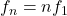where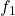is the fundamental frequency

In this problem, we know that the wave’s third harmonic has a frequency of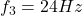This means this is the frequency for n = 3. Therefore, we can find the fundamental frequency as: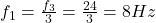Now we can also find the frequency of the 6-th harmonic using n = 6: